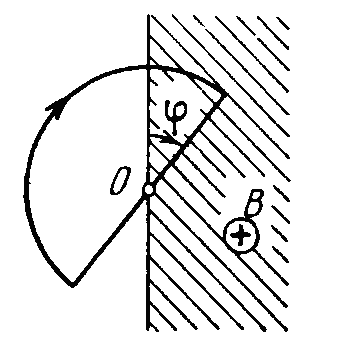# Check Our EMIA wire loop enclosing a semi-circle of radius $r = 1 \text{ m}$ is located on the boundary of a uniform magnetic field of induction $B = 2T$. At the moment $t = 0$ the loop is set into rotation with a constant angular acceleration $\alpha = 3\text{ rad/s}^{2}$ about an axis $O$ coinciding with a line of vector $B$ on the boundary. Find the magnitude emf induced (in Volts) in the loop at $t = 2\text{ sec}$.

(You can ignore self-inductance effects.)

×